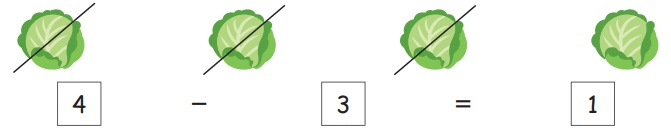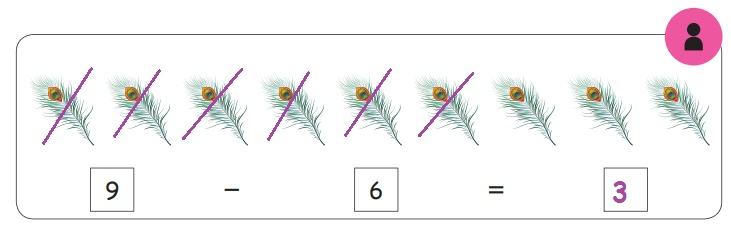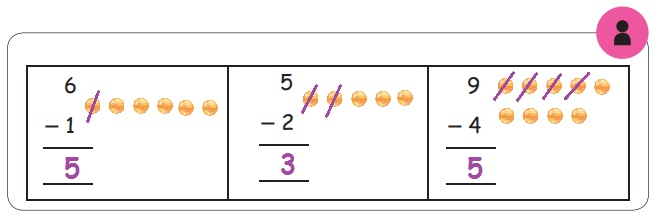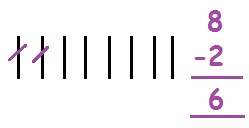Home | | Maths 1st Std | Subtraction

# Subtraction

Keywords: Take away, Left, Subtract, Difference, Less ; Teacher's note: Teacher narrates the above pictures for enhancing the vocabularies such as difference, less, move away, go away to denote subtraction.

Subtraction

Keywords: Take away, Left, Subtract, Difference, Less

Travel throughTeacher's note: Teacher narrates the above pictures for enhancing the vocabularies such as difference, less, move away, go away to denote subtraction.

Take away

Learn

Subtraction means to take away!PracticeSubtract

Learn

' −' is the sign of subtraction.PracticeLearn

Complete the subtraction fact by circling.PracticeLearn

Complete the subtraction fact by striking.PracticeSubtraction using lines

LearnPractice6 - 1 = 5

5 - 2 = 3

9 - 4 = 5

Learn5 – 1 = 4

Practice6 – 1 = 5

5 – 2 = 3

9 - 4 = 5

Try this

5-1= 4

Mind math

Fill the boxes to get 3.Mind math (Oral)

1. Pari bought 7 bananas. His brother ate 2 of them. How many bananas are left with Pari?7 – 2 = 5 bananas

2. Mani bought 6 eggs. 3 of them were broken. How many eggs are left?6 – 3 = 3 egges

3. Thendral is 8 years old. Her sister Nila is 2 years younger than her. How old is Nila?8 − 2 = 6 years old

Think like a mathematician

How can I fill these boxes using the numbers 8,5,,3 ?

8-5=3

Pleasure time

Fill the circle by + or −Try this

I am more than 5 and less than 8. I am not 7. Who am I?

Activity

Aim: Creating subtraction stories.

Things needed: Flash card with subtraction facts likeProcedure:

1. Divide the class into two groups.

2. Let the students from one group pick up the card from the deck and show it to the other group.

3. Students from that group have to make a subtraction story for that card.

4. This activity can be interchanged between the groups and continued.

Teacher's note: Teacher can encourage the children to tell stories on addition and subtraction on their own. This encourages mathematical communication in the classroom.

Tags : Numbers | Term 1 Chapter 2 | 1st Maths , 1st Maths : Term 1 Unit 2 : Numbers
Study Material, Lecturing Notes, Assignment, Reference, Wiki description explanation, brief detail
1st Maths : Term 1 Unit 2 : Numbers : Subtraction | Numbers | Term 1 Chapter 2 | 1st Maths